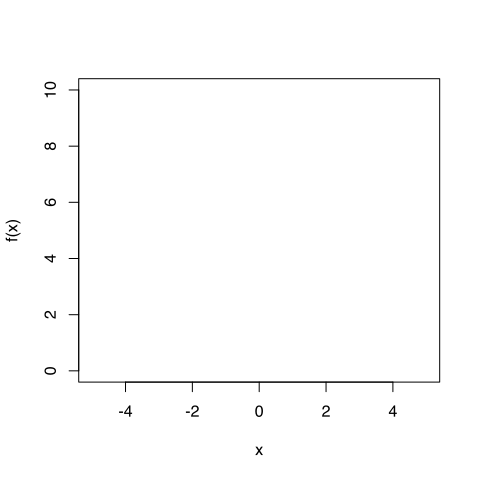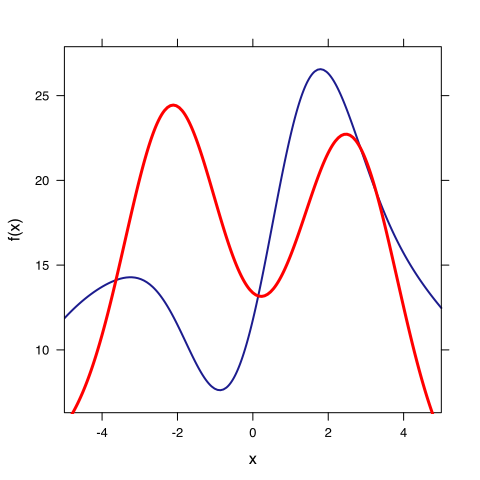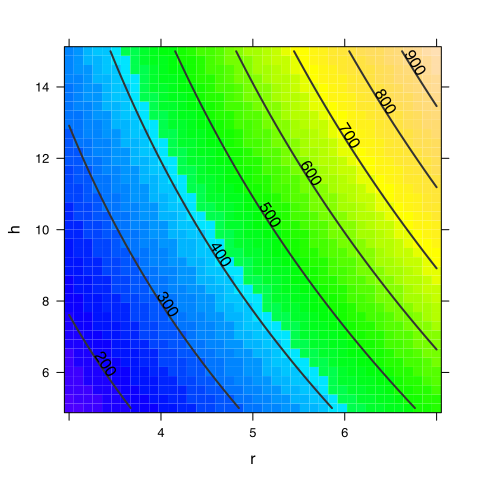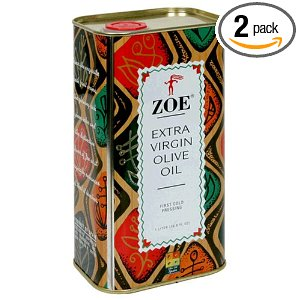# Optimization in One-Variable

Real problems involving optimization in one variable are not unheard of, but they are atypical. Careful thought should be given to the ways in which the settings and techniques being taught are misleading.

With respect to modeling, insofar as an optimization problem comes up in one or two variables, an appropriate solution technique is graphical: Look at the graph and pick the optimum.

With respect to mathematics, we should use techniques that apply in multiple variables, with the one-variable setting being used to sharpen insight.

What are the important concepts and techniques that justify teaching about optimization in one variable?

• How to search a segment efficiently.
• That the output doesn't vary much near the optimum.
• That the “indifference” to the outcome can be quantified by the second derivative.
• That local information can be used to guide meso-scale search, but you need to be careful. The virtues of a bracket.
• When one-variable optimization is likely to arise. (Not too often, except in textbooks.)
• Dealing with multiple objectives.

## A Thoroughly Unrealistic Problem

You have a function $$f(x)$$ given as an algebraic formula. Find the maximum. You're not allowed to graph the formula; just consider the derivative.

## A Somewhat Less Unrealistic Problem

You have a function $$f(x)$$ that you want to maximize. You don't have a formula for $$f(x)$$, but you can evaluate it.

Soln: Graph the function and pull out the $$x$$ that produces the maximum output.

## Blind Search for a Maximum

There is a function $$f(x)$$ that you want to maximize. You can evaluate $$f(x)$$ at any point you choose; it costs \$100,000.00 per evaluation.

What $$x$$ will you choose? How will you know when you are done?### Discovering an Algorithm for Blind Search

Insofar as we want to teach about algorithms, we should give students an easy way to generate objects on which to apply and test their algorithms. Random, smooth functions are nice for this.

As a classroom activity, construct a smooth function and let them guess:

f = rfun(~x, seed = 20120710)
f(0)

##  -4.082

f(1)

##  -4.563


Now what?

### Blind Optimization knowing derivatives

Suppose that you can compute the first and second derivatives:

df = D(f(x) ~ x)
ddf = D(f(x) ~ x & x)


Describe the search method you would use. How would you know when to stop searching?

What are the units of $$f'(x)/f'‘(x)$$? How is this quantity potentially relevant to a search?

## Teaching Optimization in a Modeling-Based Way

Often, models are used in teaching optimization in order to provide an objective function, the surface area of a tin can, the best direction to swim across a river or cross a snowy field, etc.

There are much more fundamental modeling concepts that relate to optimization. 1. The idea of an objective function, and of “policy variables”. 2. The idea of multiple incommensurate objectives. The mathematical problem of defining “best” in such a setting. 3. Attempts to deal with incommensurability by arbitrary conversion, e.g., “the value of a life.” 4. The relationship between constraints and multiple objectives.

In dealing with multiple objectives, it's important to remember that there can be no best solution and therefore automatic solution techniques are not key. Instead, the purpose of a model is often to allow people to explore alternatives and the consequences of those alternatives.

## The minimum one-variable optimization problem

You have a policy variable $$x$$ and two objective functions $$f(x)$$ and $$g(x)$$. Find the best value of $$x$$, the one that maximizes $$f(x)$$ and $$g(x)$$.## NULL


### QUESTIONS

1. What approach would you take?
2. What if you were told that the value of $$f$$ and $$g$$ are both in dollars?
3. What if $$f$$ is dollars to you and $$g$$ is dollars to the government?
4. What if $$f$$ is in billions of dollars and $$g$$ is number of lives saved?

### A TECHNIQUE

Set a constraint on one of the functions, for instance, $$g(x) \geq 20$$. Find the best value of $$f(x)$$ subject to this constraint.

Now vary the level of the constraint by a little and see how much the optimal $$f(x)$$ can improve. Was it worth it?

## A Canned Problem

### The textbook problem

“A cylindrical can is to be made to hold 1 L of oil. Find the dimensions that will minimize the cost of the metal to manufacture the can.” Stewart, Calculus: Concepts and Contexts 2/e, p. 309

### The real-world problem

We're going to sell oil in a can.

• How will it be manufactured? Cutting material, joining material. What's the cost of a straight seam compared to an edge seam? How much metal is used in the crimping of the end plate?
• How will the consumer pour from it?
• What form of opening?
• How will they handle it? Ergonomics.
• What are the failure modes? (e.g., rupture, broken seam, denting)
• What sort of label do we want?
• Is the cost of material an important issue?
• Shipping/storage issues: weight or space constrained.
• Shelf display issues.

Prices of some containers … specialtybottle.com

### The textbook mathematical problem.

• The surface area is $$A = 2 \pi r^2 + 2 \pi r h$$
• The volume is $$V = 2 \pi r^2 h$$
• “To eliminate $$h$$ we use the fact that the volume is given as 1 L, which we take to be 1000 cm3. Thus $$A® = 2 \pi r^2 + 2000/r$$.
• Differentiate, set equal to zero, and solve.
Thus, to minimize the cost of the can, the radius should be $$\sqrt{500/\pi}$$ and the height should be equal to twice the radius, namely, the diameter.''

### A Constrained Optimization Approach

• Choose $$r$$ and $$h$$.
• Plot out the area.
• Plot out the volume function.
• Solve the constrained optimization, minimizing area subject to volume.
Area = makeFun(2 * pi * r^2 + 2 * pi * r * h ~ r & h)
Volume = makeFun(2 * pi * r^2 * h ~ r & h)
plotFun(Area(r = r, h = h) ~ r & h, r.lim = c(3, 7), h.lim = c(5, 15))plotFun(Volume(r = r, h = h) ~ r & h, add = TRUE, col = "red", filled = FALSE)

## 2-D adding temporarily discontinued.

## NULL


What's the configuration that minimizes the area given a volume of 1000 cm3.

#### Extend this

An olive-oil can will be made in the shape shown in the picture.Notice that picture comes from an advertisement for a "2 pack.” There's a reason why they are selling two 1 L can instead of a single 2 L can.

For ergonomic reasons, the depth of the can should be 2.5 inches and the radius of the bend should be no less than 0.75 inches. Retail store shelves restrict the height to 12 inches. The shipping boxes must be 20 x 18 x 18 inches. Oil in contact with air will go rancid, so we want to make the top surface of the oil as small as possible. …

Construct a function giving the price of a can as a function of the policy variables. Then let the designers discuss what sorts of shapes are worthwhile. You will be able to tell them how much any given change will cost.

### Airline Pricing and Capacity

Math 135 Assignment